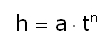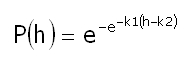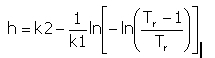﻿ Output > Rain Model

# RAIN MODEL or RAINFALL PROBABILITY

Hydro graphical Services or the competent authority is regularly measure precipitations at gaging stations ditributed in the territories of competence. calculating the inflow's data for the field measurements. Such measurements are normally published in yearly reports.

Data computation can determine the rain model for a specified recurrence interval, or finds the relation between the rain's height h and time t. Generally, for such an computation data for an extended period are required (at least 20 – 30 years).

All Rain models have this form:where a and n are constants that vary depending on the recurrence interval and are determined depending on the case.

# GUMBEL'S DISTRIBUTION

The most statical-probabilistically computation of rainfall data is that of Gumbel's, that expresses the probability that the h value might not be surpassed for a determined period. You can find it below:where, for a determined period:

P(h) = the probability that the h value might not be exceeded; (1-P) represents the  probability;

k1 and k2 = distribution parameters.

For k1 and k2 parameters estimation the moment method can be applied, with the resulting values:

k1 = 1.283/s

k2 = M – 0.450·s

Where M represents the events' average and s is the mean standard deviation.

The recurrence interval Tr represents the reversed overflow probability (1-P), that can be expressed:

Tr= 1/(1-P)

So the probability depending on the recurrence interval can be expressed: